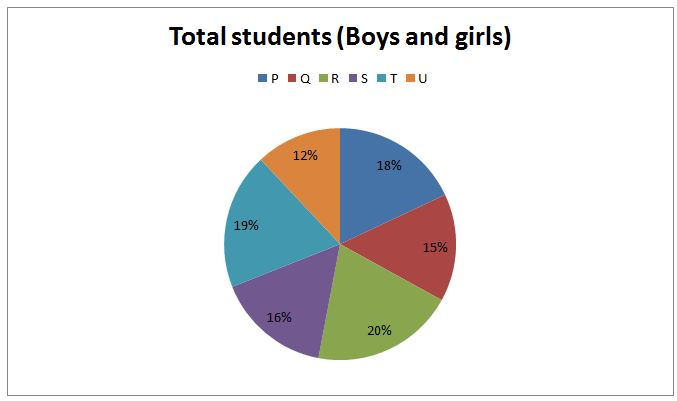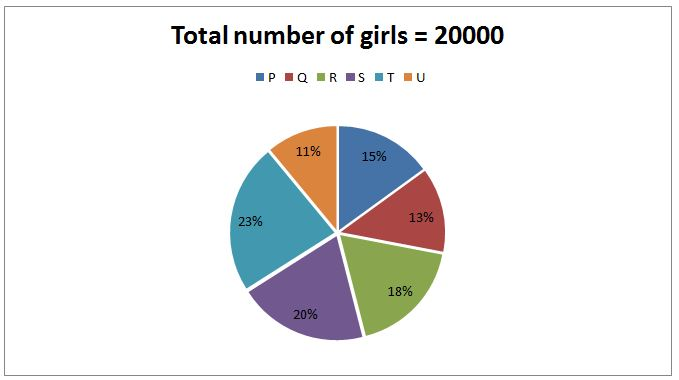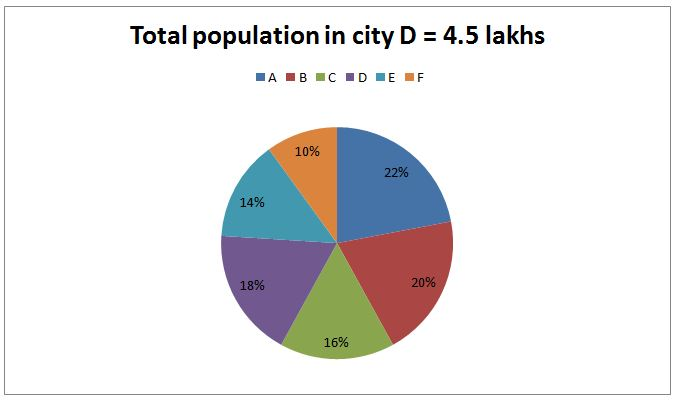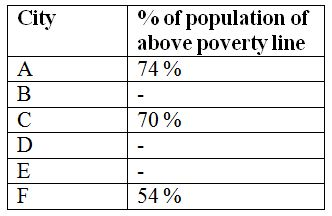# LIC AAO Mains Quantitative Aptitude Questions 2019 (Day-12)

Dear Aspirants, Our IBPS Guide team is providing new series of Quantitative Aptitude Questions for LIC AAO Mains 2019 so the aspirants can practice it on a daily basis. These questions are framed by our skilled experts after understanding your needs thoroughly. Aspirants can practice these new series questions daily to familiarize with the exact exam pattern and make your preparation effective.

[WpProQuiz 6537]

Directions (Q. 1 – 5): Study the following information carefully and answer the given questions:

The following pie chart1 shows percentage distribution of total number of students studied in six different colleges.The pie chart2 shows the percentage distribution of total number of girls studied in six different colleges.Note:

• All the questions are different from each other.

1) Find the difference between the total number of girls studied in college P and R together to that of total number of students studied in college Q, if the ratio between the total number of boys to that of girls studied in college S is 4 : 5?

a) 150

b) 250

c) 300

d) 400

e) None of these

2) Find the ratio between the total number of students studied in college R to that of total number of boys studied in college S and U together, if the total number of boys studied in college P is 2100 more than the total number of girls studied in the same college?

a) 15 : 7

b) 23 : 11

c) 59 : 43

d) 45 : 32

e) None of these

3) Total number of girls studied in college Q and S together is approximately what percentage of total number of boys studied in college R and T together, if the ratio between the total number of girls to that of boys studied in college U is 11 : 13?

a) 104 %

b) 89 %

c) 72 %

d) 56 %

e) 127 %

4) Find the average number of boys studied in college P, Q and R together, if the ratio between the total number of girls to that of boys studied in college S is 5 : 6?

a) 7150

b) 6900

c) 6650

d) 6400

e) None of these

5) Find the difference between the average number of girls studied in college P, R and S together to that from college Q, T and U together?

a) 400

b) 650

c) 500

d) 250

e) None of these

Directions (Q. 6 – 10): Study the following information carefully and answer the given questions:

The following pie chart shows the percentage distribution of total population of 6 different cities in a certain state.The following table shows the percentage of population of above poverty line in 6 different cities. Some values are missing here.6)Find the ratio between the total male population in city A to that of total female population in city F, if the ratio between the male to that of female population in city A and city F is 6 : 5 and 2 : 3 respectively?

a) 2 : 1

b) 5 : 4

c) 3 : 2

d) 7 : 5

e) None of these

7) Find the difference between the total below poverty line population in city A and B together to that of total above poverty line population in city E and F together, if the ratio between the above poverty line to that of below poverty line population in city B is 33 : 17 and the percentage of below poverty line population in city E is 32 %?

a) 50000

b) 75000

c) 60000

d) 35000

e) None of these

8) Find the average number of above poverty line population in city A, C and F together?

a) 256000

b) 262000

c) 288000

d) 274000

e) None of these

9) Total above poverty line population in city D and F together is what percentage of total female population in city B and E together, if the ratio between the male to that of female population in city B and city E is 2 : 3 and 4 : 3 respectively and the percentage of below poverty line population in city D is 38 %?

a) 84 %

b) 92 %

c) 98 %

d) 76 %

e) None of these

10) Find the difference between the average total population of city B, C and D together to that of the average above poverty line population of city A, C and F together?

a) 152000

b) 168000

c) 176000

d) 184000

e) None of these

Total number of girls studied in college S = 20000*(20/100) = 4000

Total number of students studied in college S = (4000/5)*9 = 7200

(16/100)*Total students in all the colleges = 7200

Total students in all the colleges = 7200*(100/16) = 45000

The total number of girls studied in college P and R together

= >20000*(33/100) = 6600

The total number of students studied in college Q

= >45000*(15/100) = 6750

Required difference = 6750 – 6600 = 150

Total number of girls studied in college P

= > 20000*(15/100) = 3000

Total number of students studied in college P

= > 3000 + 3000 + 2100 = 8100

(18/100)*Total number of students studied in all the colleges = 8100

Total number of students studied in all the colleges

= > 8100*(100/18) = 45000

The total number of students studied in college R

= > 45000*(20/100) = 9000

The total number of boys studied in college S and U together

= >[45000*(16/100) – 20000*(20/100)] + [45000*(12/100) – 20000*(11/100)]

= > [7200 – 4000] + [5400 – 2200]

= > 3200 + 3200 = 6400

Required ratio = 9000 : 6400 = 45 : 32

Total number of girls studied in college U = 20000*(11/100) = 2200

Total number of students studied in college U

= > (2200/11)*24 = 4800

(12/100)*Total number of students studied in all the colleges = 4800

Total number of students studied in all the colleges

= > 4800*(100/12) = 40000

Total number of girls studied in college Q and S together

= > 20000*(33/100) = 6600

Total number of boys studied in college R and T together

= >[40000*(20/100) – 20000*(18/100)] + [40000*(19/100) – 20000*(23/100)]

= > [8000 – 3600] + [7600 – 4600]

= > 4400 + 3000 = 7400

Required % = (6600/7400)*100 = 89.189 % = 89 %

Total number of girls studied in college S = 20000*(20/100) = 4000

Total number of students studied in college S

= > (4000/5)*11 = 8800

(16/100)*Total number of students studied in all the colleges = 8800

Total number of students studied in all the colleges

= > 8800*(100/16) = 55000

The total number of boys studied in college P, Q and R together

= >[55000*(18/100) – 20000*(15/100)] + [55000*(15/100) – 20000*(13/100)] + 55000*(20/100) – 20000*(18/100)]

= > [9900 – 3000] + [8250 – 2600] + [11000 – 3600]

= > 6900 + 5650 + 7400 = 19950

Required average = 19950/3 = 6650

The average number of girls studied in college P, R and S together

= >20000*(53/100)*(1/3)

The average number of girls studied in college Q, T and U together

= >20000*(47/100)*(1/3)

Required difference = 20000*(1/3)*(1/100)*[53 – 47] = 400

Directions (6-10):

The average number of girls studied in college P, R and S together

= >20000*(53/100)*(1/3)

The average number of girls studied in college Q, T and U together

= >20000*(47/100)*(1/3)

Required difference = 20000*(1/3)*(1/100)*[53 – 47] = 400

The total male population in city A

= >450000*(100/18)*(22/100)*(6/11)

The total female population in city F

= >450000*(100/18)*(10/100)*(3/5)

Required ratio = [450000*(100/18)*(22/100)*(6/11)] : [450000*(100/18)*(10/100)*(3/5)]

= > 2 : 1

The total below poverty line population in city A and B together

= >450000*(100/18)*(22/100)*(26/100) + 450000*(100/18)*(20/100)*(17/50)

= > 143000 + 170000 = 313000

The total above poverty line population in city E and F together

= >450000*(100/18)*(14/100)*(68/100) + 450000*(100/18)*(10/100)*(54/100)

= > 238000 + 135000 = 373000

Required difference = 373000 – 313000 = 60000

The average number of above poverty line population in city A, C and F together

= >[450000*(100/18)*(22/100)*(74/100) + 450000*(100/18)*(16/100)*(70/100) + 450000*(100/18)*(10/100)*(54/100)]/3

= > [407000 + 280000 + 135000]/3

= >822000/3 = 274000

Total above poverty line population in city D and F together

= >450000*(62/100) + 450000*(100/18)*(10/100)*(54/100)

= >279000 + 135000 = 414000

Total female population in city B and E together

= >450000*(100/18)*(20/100)*(3/5) + 450000*(100/18)*(14/100)*(3/7)

= > 300000 + 150000 = 450000

Required % = (414000/450000)*100 = 92 %